# Boost Converter | Step Up Chopper

DC-DC converters are also known as Choppers. Here we will have a look at the Step Up Chopper or Boost converter which increases the input DC voltage to a specified DC output voltage. A typical Boost converter is shown below.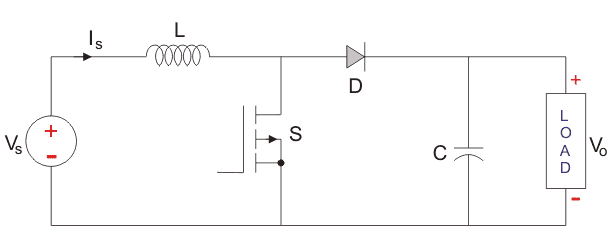The input voltage source is connected to an inductor. The solid-state device which operates as a switch is connected across the source. The second switch used is a diode. The diode is connected to a capacitor, and the load and the two are connected in parallel as shown in the figure above.

The inductor connected to input source leads to a constant input current, and thus the Boost converter is seen as the constant current input source. And the load can be seen as a constant voltage source. The controlled switch is turned on and off by using Pulse Width Modulation(PWM). PWM can be time-based or frequency based. Frequency-based modulation has disadvantages like a wide range of frequencies to achieve the desired control of the switch which in turn will give the desired output voltage. Time-based Modulation is mostly used for DC-DC converters. It is simple to construct and use. The frequency remains constant in this type of PWM modulation. The Boost converter has two modes of operation. The first mode is when the switch is on and conducting.

Mode I : Switch is ON, Diode is OFF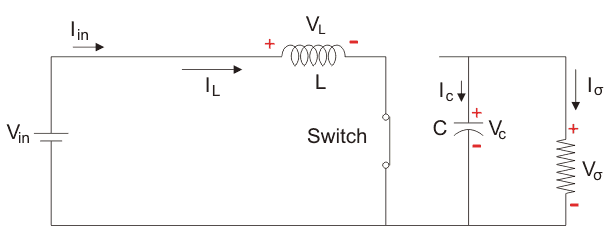The Switch is ON and therefore represents a short circuit ideally offering zero resistance to the flow of current so when the switch is ON all the current will flow through the switch and back to the DC input source. Let us say the switch is on for a time TON and is off for a time TOFF. We define the time period, T, as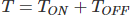and the switching frequency,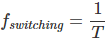Let us now define another term, the duty cycle,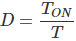Let us analyze the Boost converter in steady state operation for this mode using KVL.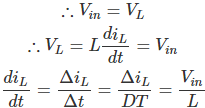Since the switch is closed for a time TON = DT we can say that Δt = DT.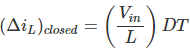While performing the analysis of the Boost converter, we have to keep in mind that

1. The inductor current is continuous and this is made possible by selecting an appropriate value of L.
2. The inductor current in steady state rises from a value with a positive slope to a maximum value during the ON state and then drops back down to the initial value with a negative slope. Therefore the net change of the inductor current over anyone complete cycle is zero.

Mode II : Switch is OFF, Diode is ON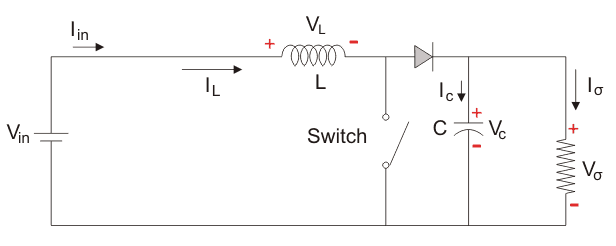In this mode, the polarity of the inductor is reversed. The energy stored in the inductor is released and is ultimately dissipated in the load resistance, and this helps to maintain the flow of current in the same direction through the load and also step-up the output voltage as the inductor is now also acting as a source in conjunction with the input source. But for analysis, we keep the original conventions to analyze the circuit using KVL.

Let us now analyse the Boost converter in steady state operation for Mode II using KVL.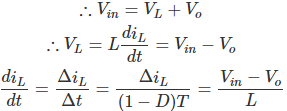Since the switch is open for a time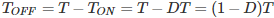we can say that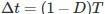.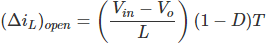It is already established that the net change of the inductor current over any one complete cycle is zero.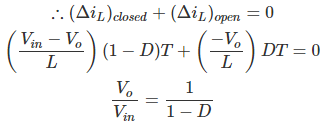We know that D varies between 0 and 1. But as we can see from the equation above that if D = 1 then the ratio of output voltage to input voltage at steady state goes to infinity, which is not physically possible. In fact as the Boost converter is a non-linear circuit, in a practical Boost converter the duty cycle, D, if kept at a value greater than 0.7 will lead to instability. A circuit of a Boost converter and its waveforms are shown below. The inductance, L, is 20mH and the C is 100µF and the resistive load is 20Ω. The switching frequency is 1 kHz. The input voltage is 100V DC and the duty cycle is 0.5.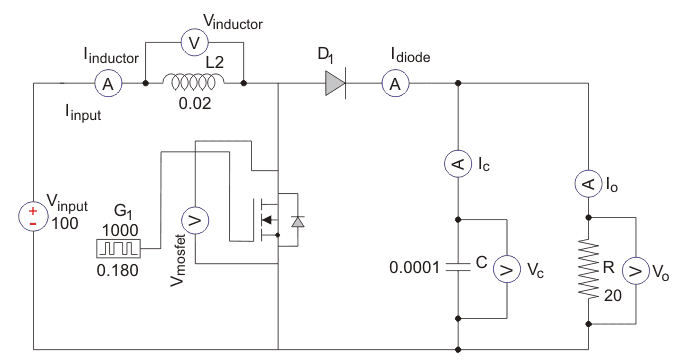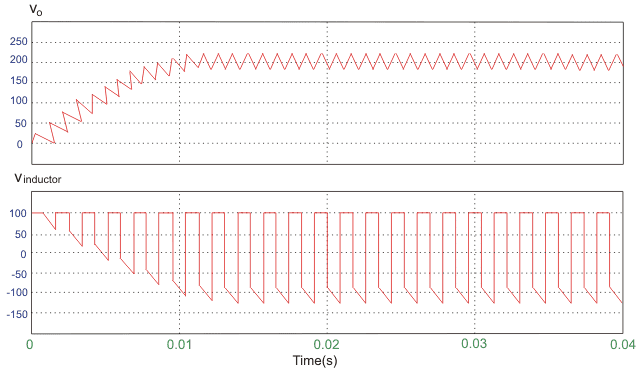The voltage waveforms are as shown above and the current waveforms are as shown in the figure below.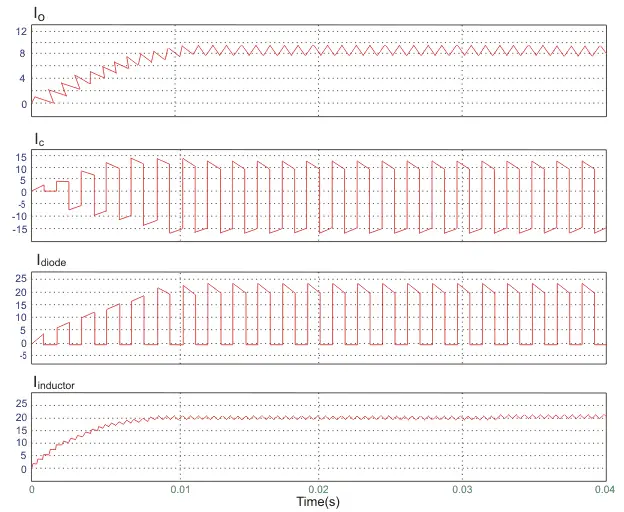Want To Learn Faster? 🎓
Get electrical articles delivered to your inbox every week.
No credit card required—it’s 100% free.Electrical4U is dedicated to the teaching and sharing of all things related to electrical and electronics engineering.

### 2 thoughts on “Boost Converter | Step Up Chopper”

1.thank you love the information

•